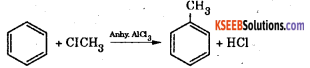# 1st PUC Chemistry Question Bank Chapter 13 Hydrocarbons

## Karnataka 1st PUC Chemistry Question Bank Chapter 13 Hydrocarbons

### 1st PUC Chemistry Hydrocarbons One Mark Questions and Answers

Question 1.
What are hydrocarbons ?
Organic compounds containing only carbon and hydrogen are called hydrocarbons.

Question 2.
What are alkanes ?
Alkanes are saturated aliphatic hydrocarbons containing carbon to carbon single bonds.

Question 3.
Give the general formula of alkanes.
The general formula of alkanes is CnH2n+2

Question 4.
What are saturated hydrocarbons ?
The hydrocarbons containing only carbon – carbon single bonds saturated hydrocarbons.

Question 5.
What is the order of reactivity of halogens towards alkanes.
The reactivity of halogens towards alkanes varies as Flourine > Chlorine > Bromine > Iodine i.e., F > Cl > Br > I.Question 6.
Why alkanes are called parafins ?
Because of low reactivity.

Question 7.
What is the nature ofC-C bond in alkanes?
These are sigma (σ) bonds.

Question 8.
What type of structural isomerism is shown by alkanes?
Chain Isomerism.

Question 9.
What is Photochemical reaction ?
Reaction carried in the presence of light.

Question 10.
Which class of organic compounds are potential carcinogen?
Polynuclear aromatic hydrocarbons.

Question 11.
What is huckel Rule ?
It states that a compound is said to be aromatic. If it has (4n +2) p electrons which are de-localised where n = 0,1, 2, 3, ………

Question 12.
Name the scientist who first isolated benzene.

Question 14.
What is decarboxylation ?
The process of removing carbon dioxide from sodium salts of acid with the help of soda lime.

Question 15.
What is electrophilic subsitution reaction ?
Those reaction in which weaker electrophile is replaced by stronger electrophile are called electrophilic substitution reaction.

Question 16.
Why is addition reaction of bromine to benzene difficult?
Due to delocalisation of π electrons. It does not have pure double bond.

Question 17.
What are arenas? Give general formula of Monocyclic arenas.
Arenes are aromatic hydrocarbons. General formula of arenas is CnH2n-6

Question 18.
What are unsaturated hydrocarbons?
The hydrocarbons which contain atleast one carbon to carbon double bond or triple bond are called unsaturated hydrocarbons.

Question 19.
What type of isomerism is shown by alkenes ?
Chain isomerism.

Question 20.
What are alkenes ?
Alkenes are unsaturated aliphatic hydrocarbons containing one carbon to carbon double bond.Question 21.
Give the general formula of alkenes.
The general formula of alkenes is CnH2n.

Question 21.
Why are alkenes and alkenes called unsaturated hydrocarbons?
Due to presence of C = C & C ≡ C bonds respectively.

Question 22.
What is the state of hybridisation of carbon atoms in alkenes ?
Sp2

Question 23.
A compound decolourise yellow colour of bromine. What does it show ?
It is unsaturated in nature.

Question 24.
What is Lindlar’s catalyst ?
Palladium supported over calcium carbonate and deactivated with quinoline is called Lindlar’s Catalyst.

Question 25.
Name the hydrocarbon which contains acidic hydrogen.
Ethylene (HC ≡ CH).

Question 26.
Give one hydrocarbon which delcolourises alk KMnO4.
Ethene (CH2 = CH2)

Question 27.
Alcoholic potash.

Question 28.
What is the major product formed when propene reacts with hydrogen bromide ?
2 – bromopropane.

Question 29.
Name the product formed when HBr reacts with 2 – methyl propene.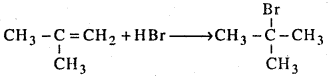Question 30.
What are alkynes?
Alkynes are unsaturated aliphatic hydrocarbons containing one carbon to carbon triple bond.

Question 31.
Give the general formula of alkynes.
The general formula of alkynes is CnH2n-2

Question 32.
What is the hybridisation of carbon atoms in acetylene ?
sp

Question 33.
What is a vicinal dihalide ?
A compound with halogen atoms on adjacent carbon atom.

Question 34. Give the example of vicinal dihalide.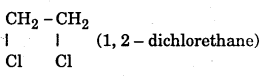Question 35.
Give an example of geminal dihalide.
CH3 – CHCl2 (1,1 – dichlorethane)Question 36.
Give an example of alkyne.
Acetylene CH ≡ CH

Question 37.
Why are alkenes called olefins ?
Because they are oily forming compounds i.e., most of the oils are unsaturated.

Question 38.
Give an addition reaction of alkynes.
CH ≡ CH + HBr → CH2 ≡ CH – Br (Vinyl Bromide)

Question 39.
Give the resonance structures of benzene.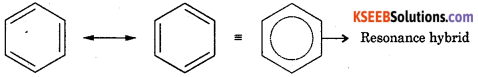Question 40.
Name the electrophiles in the following reactions

1. Chlorination
2. Nitration
3. Sulphonation
4. Friedel Craft’s methylation

1. Chlorination – Cl+
2. Nitration – $$\mathrm{NO}_{2}^{+}$$
3. Sulphonation – SO3 .
4. Friedel Crafts methylation – $$\mathrm{CH}_{3}^{+}$$

Question 41.
Write the Geometrical isomers of CHBr = CHBr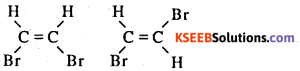Question 42.
Which catalyst is most effective even in the polymerization of ethane to polythene ?
Ziegler – Nata Catalyst

Question 43.
What is the compostion of Zeigler Natta Catalyst?
Triethylaluminium and titanium tetracholride inert solvent.

Question 44.
Name one Carcinogenic compound.
Anthracene of Benzanthracene.

Question 45.
What is the number of a and n bonds in N ≡ C-CH = CH- C ≡ N?
There are 7 σ bonds and 5 π bonds.

Question 46.
Name the compound formed when heptane is subjected to aromatisation.
Toulene.

Question 47.
What is the cause of Geometrical Isomerism ?
The restricted rotation around carbon to carbon double bond

Question 48.
What is cracking of hydrocarbon ?
Cracking is a process of breaking higher hydrocarbons by heating.

Question 49.
What is the trade name of Benzene Hexacarbon ?
Gammaxane.### 1st PUC Chemistry Hydrocarbons Two Marks Questions and Answers

Question 1.
What is the action of ethane with bromine in carbon tetra chloride?
Ethene reacts with bromine in carbon tetrachloride to give 1, 2 – dibromethane. Orange colour of bromine is discharged.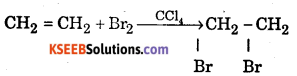Question 2.
What is the action of hydrogen halide on alkene?
Alkene reacts with hydrogen halide to form alkyl halide. The addition is governed by Markownikoff’s rule.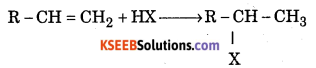Question 3.
How do you obtain an alkene from alkyne ?
Alkynes react with hydrogen in the presence of (Palladium supported over calcium carbonate and deactivated with quinoline) Lindlar’s catalyst, to give alkene.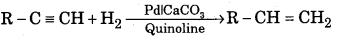Question 4.
How do you convert ethene to ethane?
When a mixture of ethene and hydrogen is passed over heated nickel catalyst at 200°C, ethane is obtained.
$$\mathrm{H}_{2} \mathrm{C}=\mathrm{CH}_{2}+\mathrm{H}_{2} \frac{\mathrm{Ni}}{200^{\circ} \mathrm{C}} \mathrm{CH}_{3}-\mathrm{CH}_{3}$$

Question 5.
How is propene obtained from propyl chloride ?
When propyl chloride is heated with alcoholic potash propene is obtained.
$$\mathrm{CH}_{3}-\mathrm{CH}_{2}-\mathrm{CH}_{2} \mathrm{Cl}+\mathrm{KOH}_{(\mathrm{alc})} \stackrel{\Delta}{\longrightarrow} \mathrm{CH}_{3}-\mathrm{CH}=\mathrm{CH}_{2}+\mathrm{KCl}+\mathrm{H}_{2} \mathrm{O}$$.

Question 6.
What is Wurtz reaction ? Give example.
When alkyl halides are heated with sodium metal in ether medium higher alkanes are formed. This reaction is known as Wurtz reaction and employed for the synthesis of higher alkanes containing even number of carbon atoms.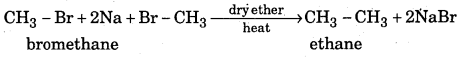Question 7.
How is Methane prepared from sodium acetate ?
Decarboxylation : When sodium salt of carboxylic acid is heated with soda lime (mixture of sodium hydroxide and calcium oxide), an alkane containing one carbon atom less than parent carboxylic acid is formed. This reaction is called decarboxylation.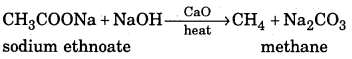Question 8.
How is alkane prepared from Kolbe’s electrolytic method ?
A concentrated sodium salt of a carboxylic acid is electrolysed. The alkane is formed at the anode as one of the products. This process is called Kolb’e electrolysis.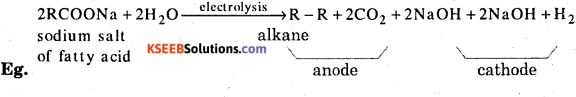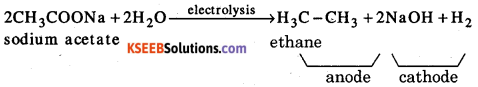Question 9.
What is Pyrolysis ? Give example.
The decomposition of higher alkane into a mixture of lower alkanes, alkenes, etc by the application of heat is called pyrolysis or cracking.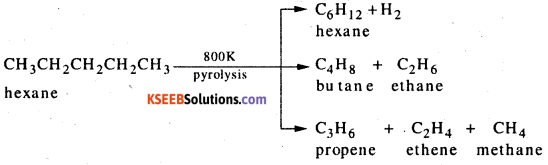Question 10.
What is Aromatisation ? Give example.
Hexane or higher akanes when heated in presence of vanadium pentoxide (V2O5), molybdenium oxide (MO2O3) or chromium oxide (Cr2O3) supported on alumina (Al2O3) at 800 K and 10-20 atm pressure give benzene or its alkyl derivatives with the liberation of hydrogen. This dehydrogenation process which involves cyclis^ion of alkanes is known as aromatization.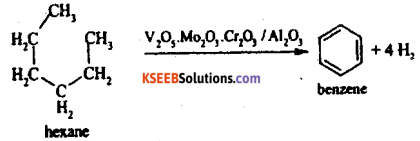Question 11.
What is isomerisation ? Give example.
When unbrached alkanes are heated with anhydrous aluminium chloride and hydrogen chloride, isomeric branched alkanes are formed. This process is called isomerisation.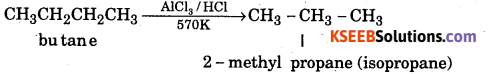Question 12.
What is Geometrical isomerism ?
Compounds having same molecular formula and structural formula but differ in the spatial arrangement of atoms or group of atoms due to restricted rotation of carbon carbon double bond.

Question 13.
What are the conditions for Geometrical isomerism ?

• The molecule should contain double bond.
• Each carbon atom joined by the double bond, must be attached to two different groups.

Question 14.
What is Ozonalysis ? Give example.
The ozonolysis of alkenes involves addition of ozone molecule to carbon-carbon double bond of alkenes to form ozonide. This follows hydrolysis of the ozonoide by H2O/Zn to yield corresponding aldehydes and / or ketones. The addition of ozone to alkene followed by hydrolysis is known as ozonolysis.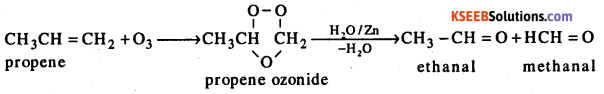Question 15.
How is ethene prepared ?
When 1, 2 – dihaloalkanes (vicinal dihalides) are heated with zinc metal in presence of methanol corresponding alkenes are formed. This reaction is called dehalogenation.
$$\mathrm{Br}-\mathrm{CH}_{2}-\mathrm{CH}_{2}-\mathrm{Br}+\mathrm{Zn} \frac{\mathrm{CH}_{3} \mathrm{OH}}{\text { heat }} \mathrm{CH}_{2}=\mathrm{CH}_{2}+\mathrm{ZnBr}_{2}$$Question 16.
What are the uses of LDPE and HDPE ?
LDPE is used for

• making transparent films for packaging garments and food,
• as an insultor.

HDPE is used for

• making crates and large boxes
• production of carry bags, containers, house hold articles and toys.

Question 17.
What happens when Ammonical solution of silver Nitrate treated with Acetylene ?
Acetylene when passed through an ammonical solution of silver nitrate (Toallen’s reagent) white solid of silver acetylide is separated.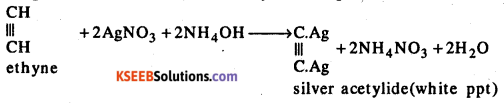Question 18.
Give four examples for isomerism in Arenes.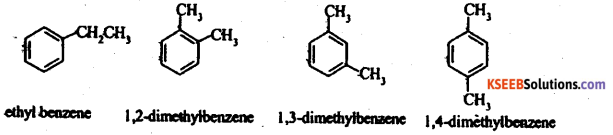Question 19.
Give three examples for fused polycyclic arene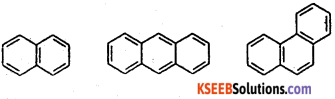Question 20.
State Markownkoff s rule.
Answer: When an unsymmetrical molecule adds to an unsymmetrical alkene, the negative part of the adding molecule adds to the carbon atom involved in the double bond, containing the least number of hydrogen atoms. Thus according to Markonwkoff’s rule the addition takes place as follows :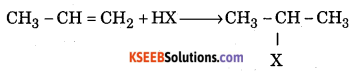### 1st PUC Chemistry Hydrocarbons Four/Five Mark Questions and Answers

Question 1.
Explain the action of chlorine on methane in the presence of sunlight.
Methane reacts with chlorine in the presence of diffused sunlight to give methyl chloride, methylene chloride, chloroform and carbon tetrachloride.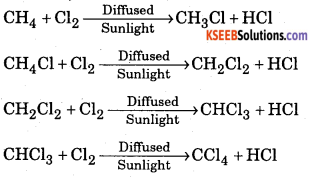Question 2.
Explain the mechanism of chlorination of methane, according to Free Radical.
Mechanism of chlorination of methane involves three types.
1. Initiation : Chlorine absorbs energy and undergoes homolysis to give chlorine free radicals.
$$\mathrm{Cl}-\mathrm{Cl} \stackrel{\mathrm{hy}}{\longrightarrow} 2 \mathrm{Cl}^{\bullet}$$

Step 2 : Propagation : Chlorine free radical reacts with methane to give methyl free radical.
$$\mathrm{Cl}^{\bullet}+\mathrm{CH}_{4} \longrightarrow \mathrm{CH}_{3}^{\bullet}+\mathrm{HCl}$$
The methyl free radical reacts with chlorine to form methyl chloride and chlorine free radical.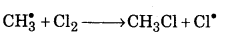Step 3 : Termination : Free radials combine to form stable products.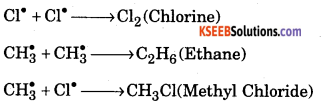Question 3.
Explain the mechanism of addition of hydrogen bromide to propene.
The mechanism of addition of hydrogen bromide to propene takes place in three steps. Step 1: Hydrogen bromide dissociates into H+ and Br-
H-Br → H++Br

Step 2 : The electrophile H+ attacks propene to form carbocation.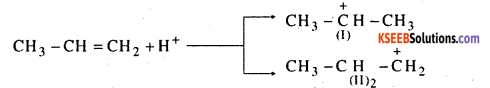of the two carbocations (I) and (II), carbocations (I) is more stable and is formed more readily.

Step 3 : The nucleophile Br attacks the carbocation (I) to give 2 – bromopropane.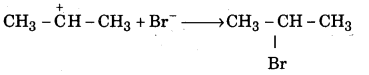Question 4.
How is the manufacture of LDPE & HDPE ?
Low density polythene is manufactured by compressing ethane under very high pressure (1500-2000 atmospheres) at about 475 K in the presence of a small amount of oxygen as catalyst.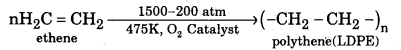High density polythene is obtained by passing ethene under pressure of 6 atmospheres at about 340 K into heptane, an inert solvent containing Zielgler-Natta catalyst (triethyl) aluminium and titanium tetrachloride)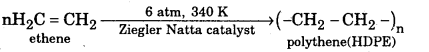Question 5.
Give four uses of aceylene.

1. Oxyacetylene flame is used for cutting and welding of metals.
2. Acetylene and its derivatives are widely used in synthetic organic chemistry for synthesis of cis and trans-alkenes, methyl ketones, etc.
3. Acetylene is used as illuminant in hawker’s lamp and in light houses.
4. Acetylene is used for ripening of fruits and vegetables.
5. Acetylene is used for manufacture of ethyl alcohol, acetaldehyde, acetic acid, vinyl plastics, synthetic rubbers such as Buna-N and synthetic fibres such as Orlan.

Question 6.
Give 4 uses of ethene.

1. Lower members of the family are used as fuels and illuminants.
2. Alkenes and substituted alkenes upon polymerization form a number of useful polymers such as polythene, PVC, Teflon, orlon, etc.,
3. Ethene is employed for preparation of ethyl alcohol and ethylene glycol (anti-freeze).
4. Ethylene is used for artificial ripening of green fruits.
5. Ethylene also used in oxygen-ethylene flame for cutting and welding of metals.

Question 7.
Give four uses of methane.

1. Methane in the form of natural gas (CNG) is used for running scooters, cars, buses, etc., LPG (Mixture of butane and isobutene) is used as a fuel in homes as well as in industry.
2. Methane is used to make carbon black which is used in the manufacture of printing inks, paints and automobile tyres.
3. Cataltic oxidation of alkanes gives alcohols, aldehydes an carboxylic acids.
4. Higher alkanes in form of gasoline, kerosene oil, diesel, lubricating oils and paraffin wax are widely used.
5. Methane is used for manufacture halogen containing compounds such as CH2Cl2 , CHCl3 , CCl4 etc. which are used as solvents both in laboratory and industry.

Question 8.
Give the structural elucidation of benzene.
1. From elemental analysis and molecular mass determination, the molecular formula of benzene is found to be C6H6.

2. On the basis of molecular formula benzene is highly unsaturated compound.

3. Benzene does not show alkene properties. Benzene is quite stable. It does not decolourise cold aqueous solution of potassium paramanganate.

4. Benzene shows cyclic structure,
(a) Benzene contains three double bonds. Benzene adds three moles of hydrogen in presence of nickel catalyst to form cyclohexane.

$$\mathrm{C}_{6} \mathrm{H}_{6}+3 \mathrm{H}_{2} \frac{180^{\circ} \mathrm{C}}{\mathrm{Ni}} \mathrm{C}_{6} \mathrm{H}_{12}$$

(b) All the six hydrogen atoms in benzene are identical – benzene reacts with bromine in presence of FeBr3 catalyst to form monobromobenzene.

$$\mathrm{C}_{6} \mathrm{H}_{6}+\mathrm{Br}_{2} \stackrel{\mathrm{FeBr}_{3}}{\longrightarrow} \mathrm{C}_{6} \mathrm{H}_{5} \mathrm{Br}+\mathrm{HBr}$$

5. Kekule proposed benzene is a planar ring structure with alternate single and double bonds.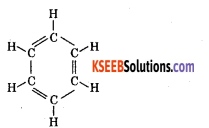6. To overcome this drawback, Kekule suggested that benzene is a mixture of two forms, which are in rapid oscillation.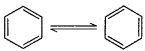7. It is found that the bond length in benzene is same for all C-C bonds (0.139 nm). This lies between C-C single bond (0.154 nm) and C-C double bond length (0.134 nm).

8. Resonance structure: Resonance hybrid is more stable than structure 1 and 2. The stability of benzene due to resonance is so great that n bonds of the molecule will normally resist breaking. This explain the stability of benzene.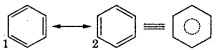Question 9.
Explain the mechanism of halogenation or chlorination of benzene.
Halogenation: Benzene reacts with chlorine in the presence of FeCl3 or AlCl3 to form chlorobenzene.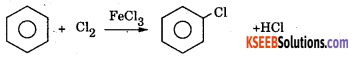Machanism : This involves the following steps.

Step 1: Generation of eiectrohile Cl – Cl + FeCl3 → Cl+ + $$\mathrm{FeCl}_{4}^{-}$$
Step 2 : The electrophile Cl+ attacks benzene ring to form a carbon cation which is resonance stabilised.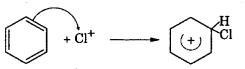Step 3 : Loss of a proton to give chlorobenzene. The proton is removed by FeCU.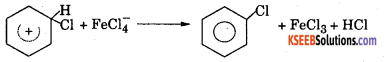Question 10.
Explain the mechanism of nitration of benzene.
Nitration benzene reacts with a mixture of concentrated nitric acid and concentrated sulphuric acid at 50°C to form nitrobenzene.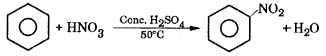Mechanism : This involves the following steps.
Step 1: Generation of electrophile nitronium ion $$\mathrm{NO}_{2}^{+}$$
$$\mathrm{HNO}_{3}+2 \mathrm{H}_{2} \mathrm{SO}_{4} \longrightarrow \mathrm{NO}_{2}^{+}+\mathrm{H}_{3} \mathrm{O}^{+}+2 \mathrm{HSO}_{4}^{-}$$

Step 2 : The electrophile NO2 attacks the benzene ring to form a carbocation which is resonance stabilized.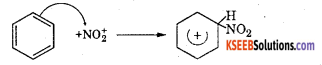Step 3 : Loss of a proton to give nitrobenzene. The proton is removed by $$\mathrm{HSO}_{4}^{-}$$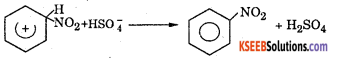Question 11.
Explain the mechanism of sulphonation of benzene?
Sulphonation : Benzene reacts with concentrated sulphuric acid at 80°C to form benzene sulphoric acid.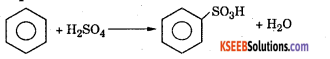Mechanism : This involves the following steps.

Step 1: Generation of electrophile SO3.
$$2 \mathrm{H}_{2} \mathrm{SO}_{4} \rightleftharpoons \mathrm{SO}_{3}+\mathrm{H}_{3} \mathrm{O}^{+}+\mathrm{HSO}_{4}^{-}$$

Step 2 : The electrophile SO3 attacks the benzene ring to form a carbocation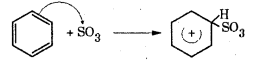Step 3: Loss of a proton to from a sulphonate ion. The proton is removed by $$\mathrm{HSO}_{4}^{-}$$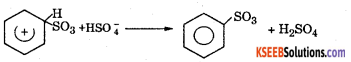Step 4 : Addition of proton to give benzene sulphonic acid.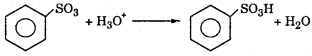Question 12.
Explain the mechanism of Friedel craft alkylation of benzene.